# Collection of Engineering Photos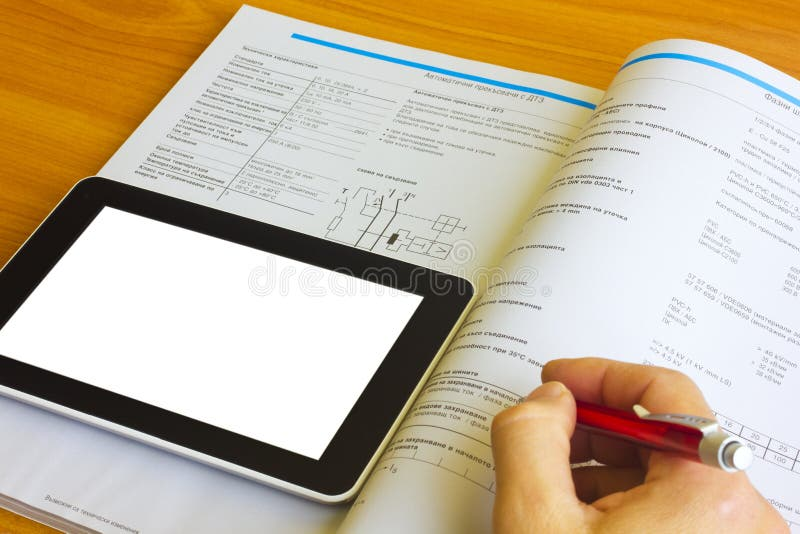Tablet computer over engineering journalTablet computer over engineering journal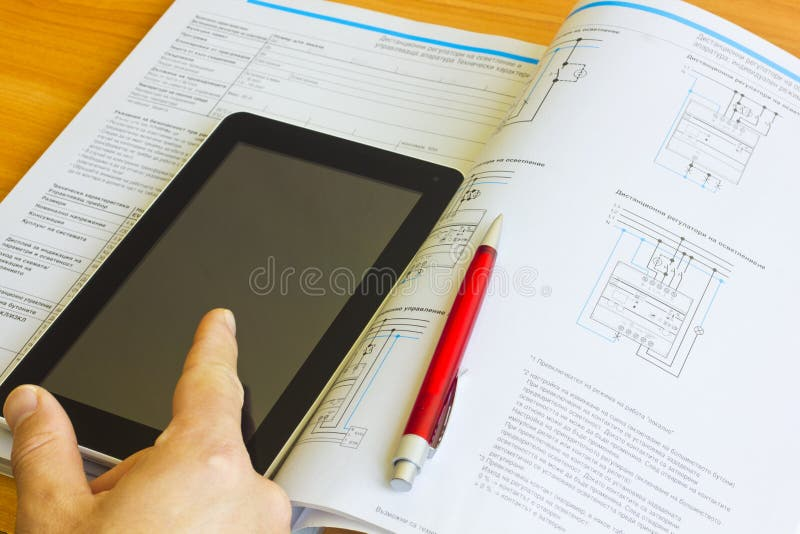Tablet computer over engineering journalTablet computer over engineering journal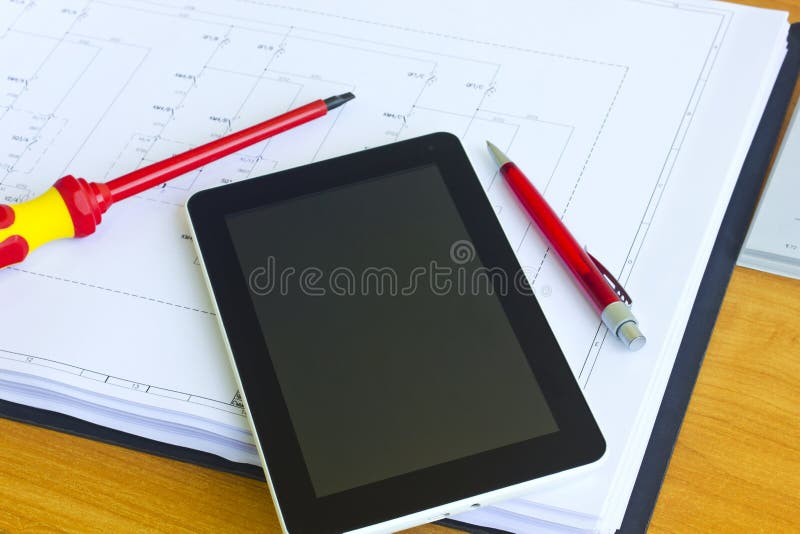Tablet over engineering diagram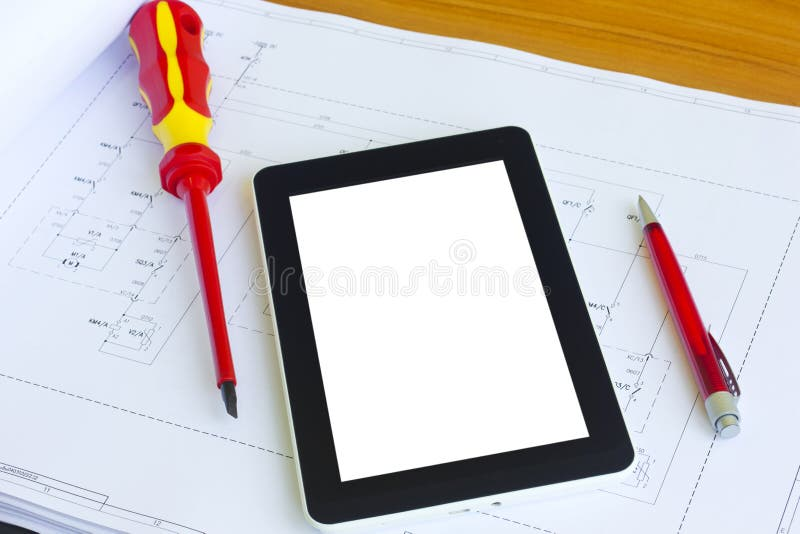Tablet over engineering diagramTablet over engineering diagram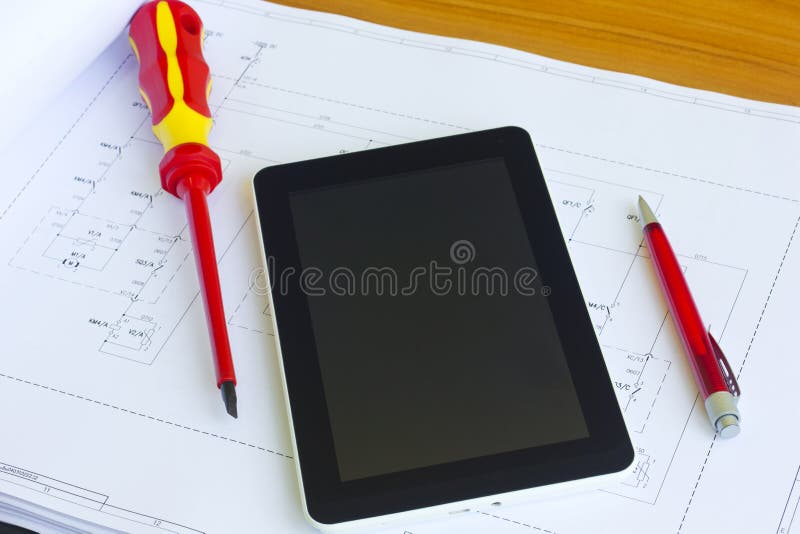Tablet over engineering diagram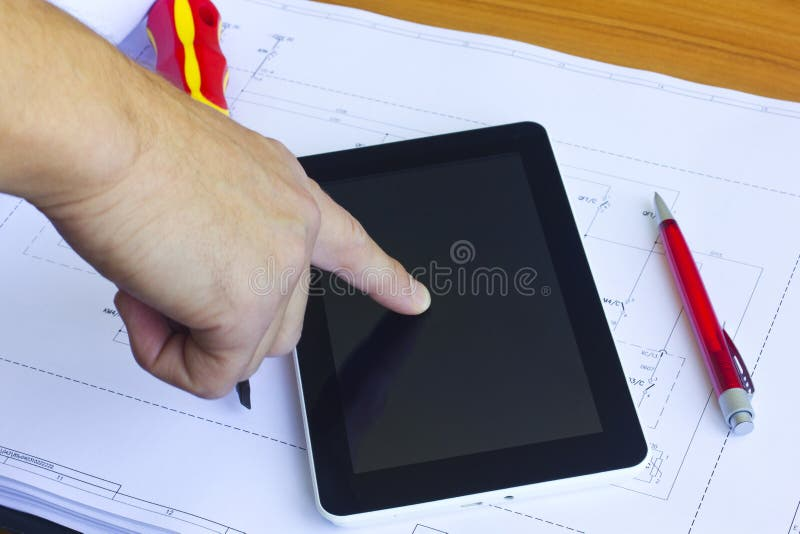Tablet over engineering diagram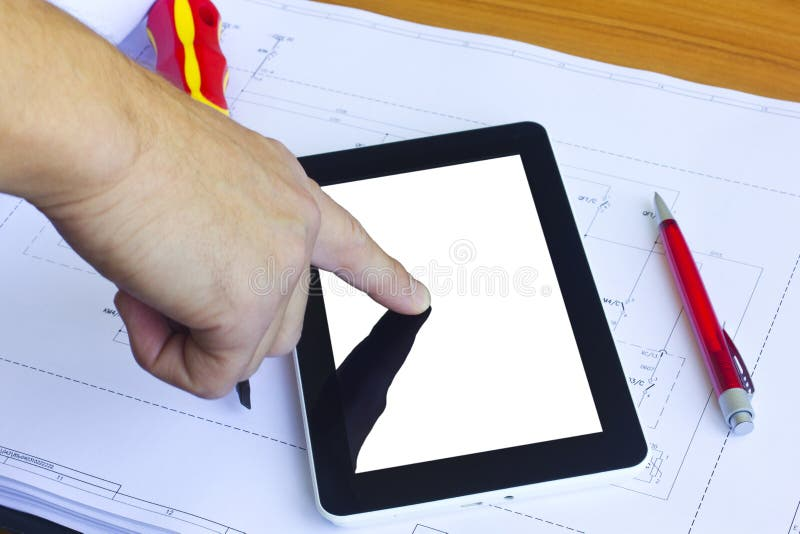Tablet over engineering diagram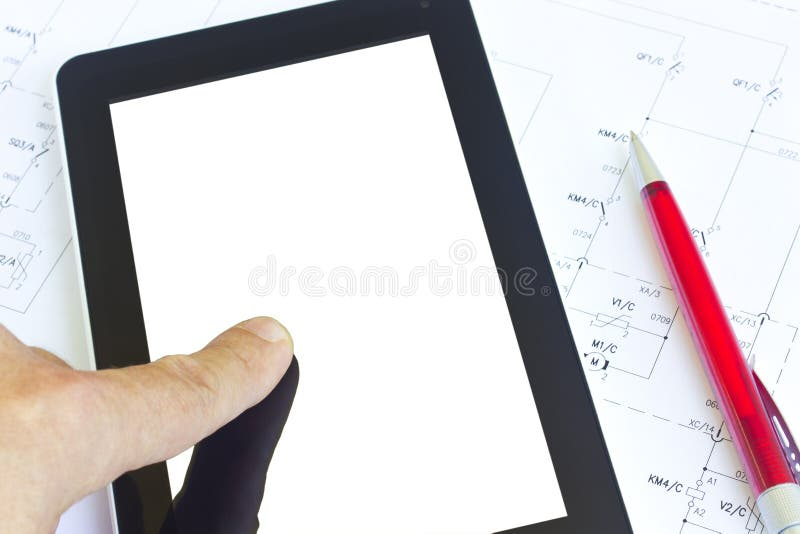Tablet over engineering diagram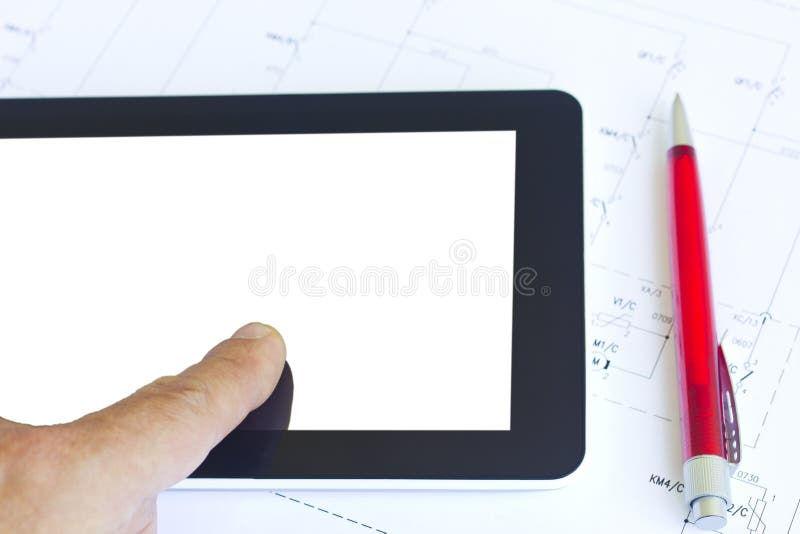Tablet over engineering diagram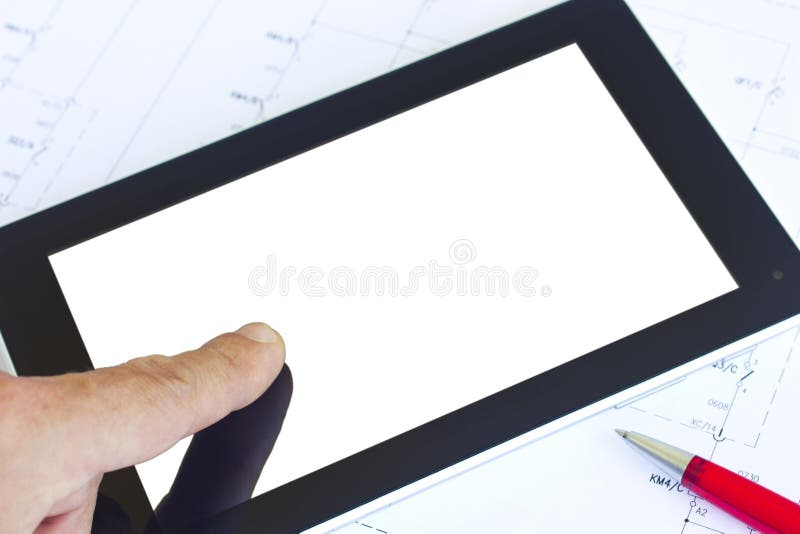Tablet over engineering diagram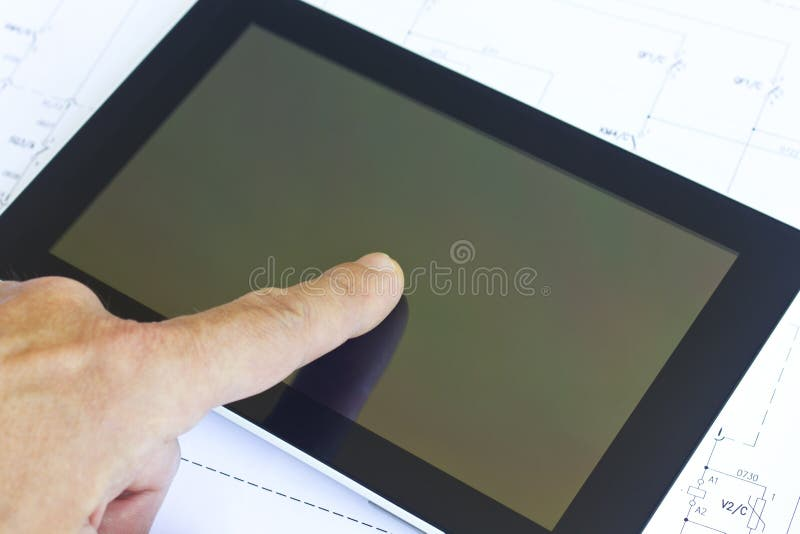Tablet over engineering diagram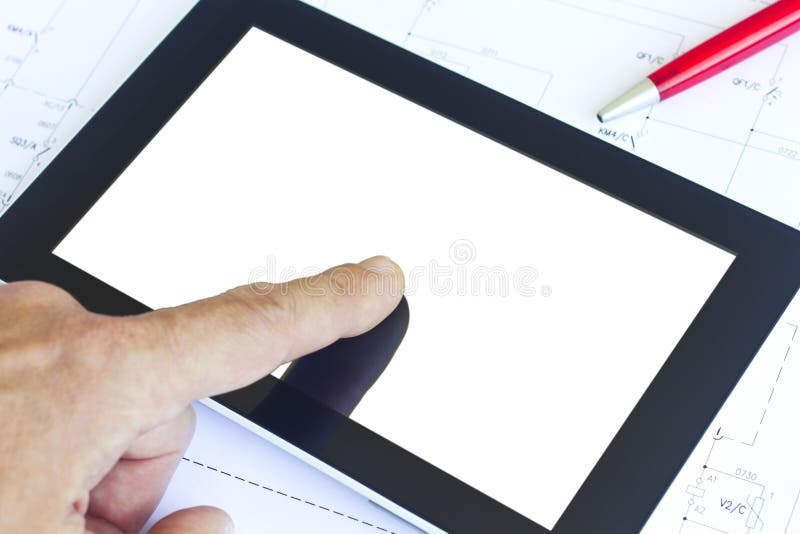Tablet over engineering diagram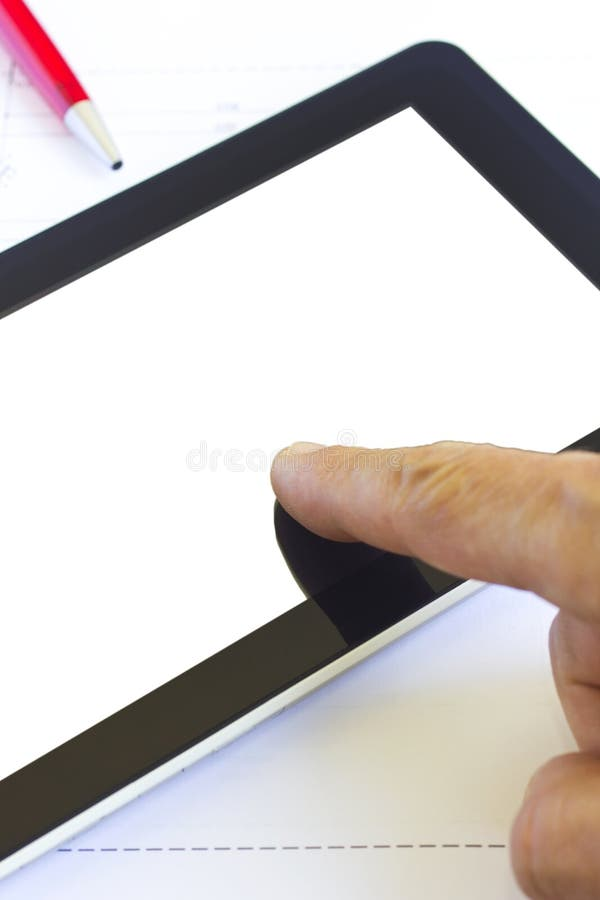Tablet over engineering diagram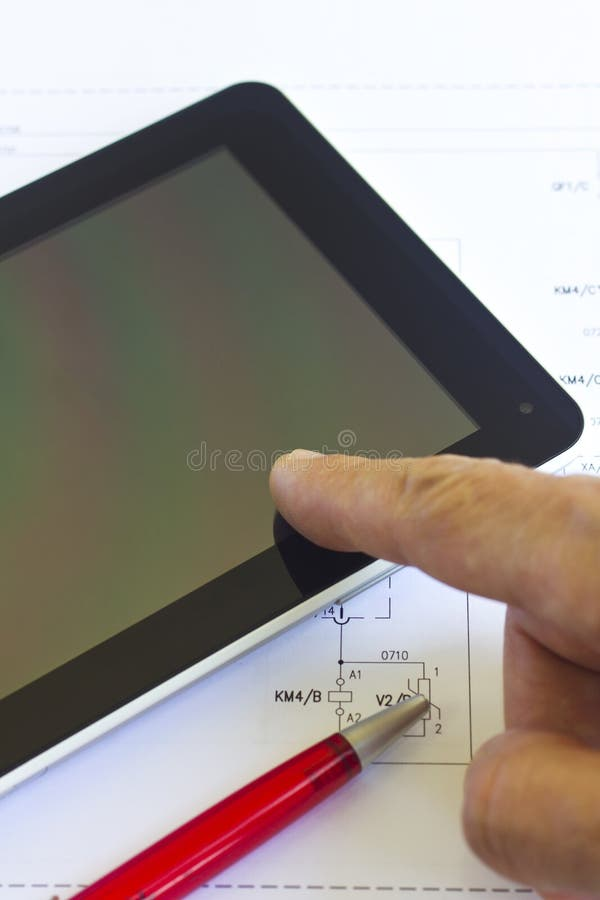Tablet over engineering diagram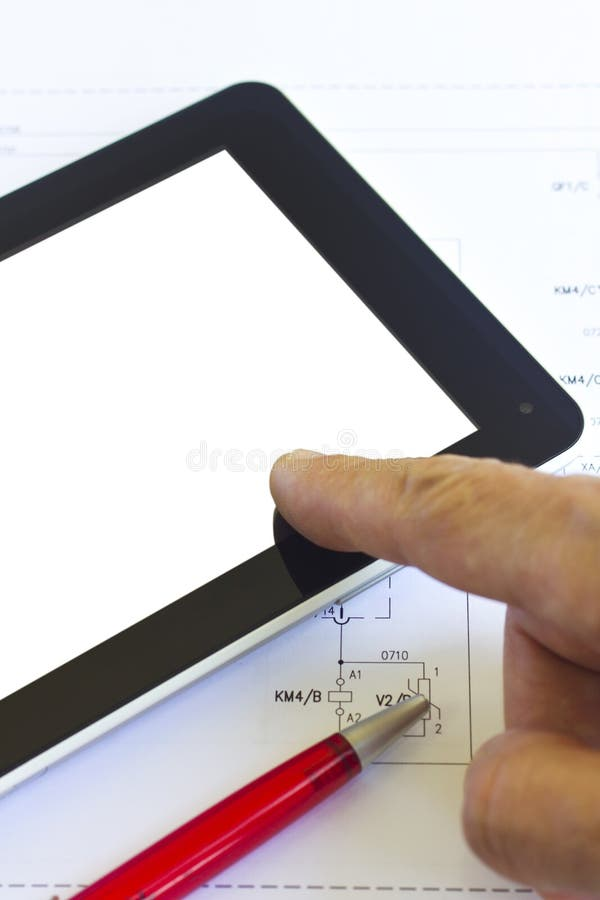Tablet over engineering diagram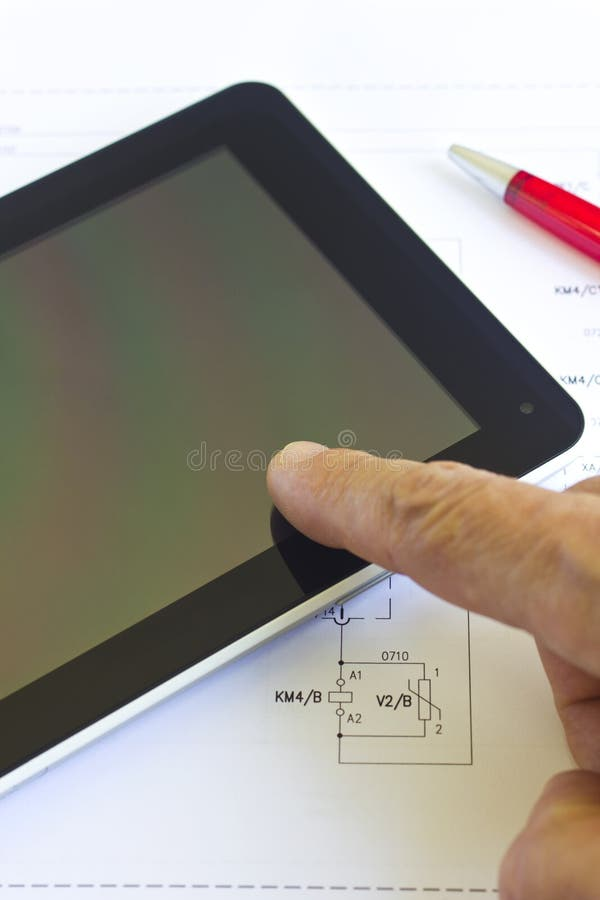Tablet over engineering diagram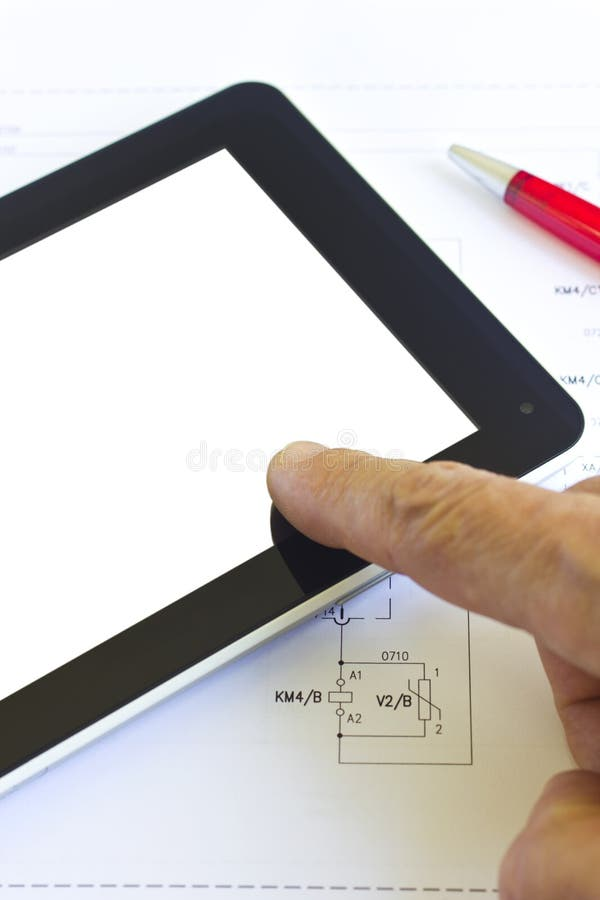Tablet over engineering diagram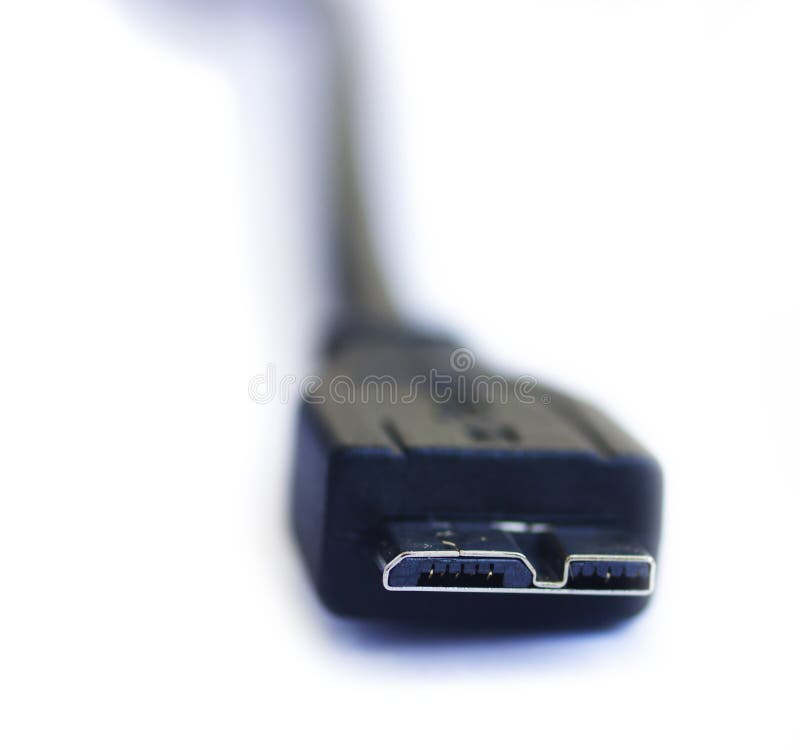USB3.0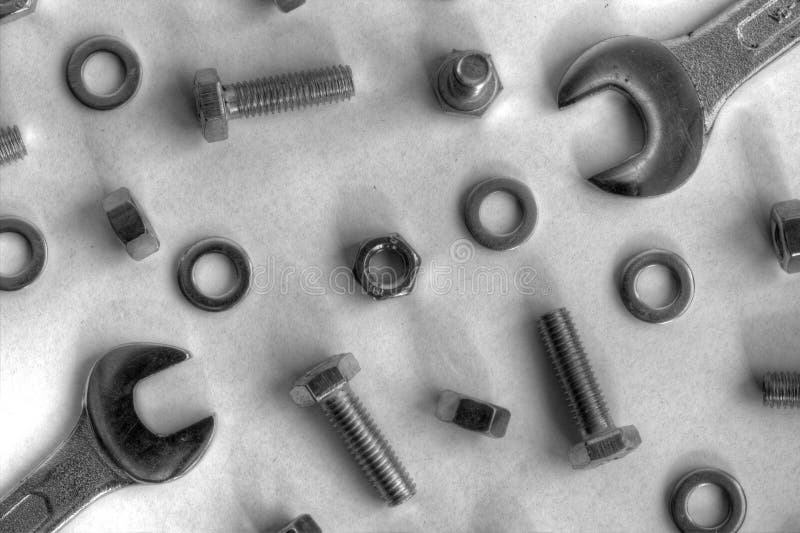Metal works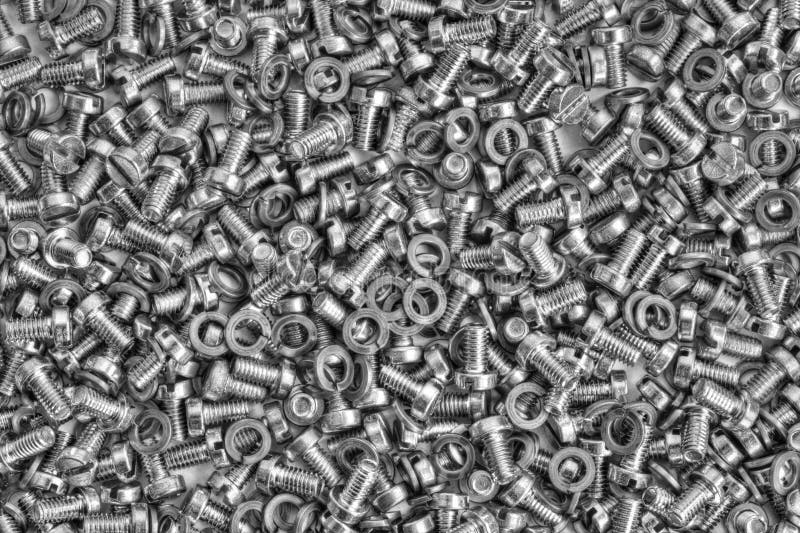Metal background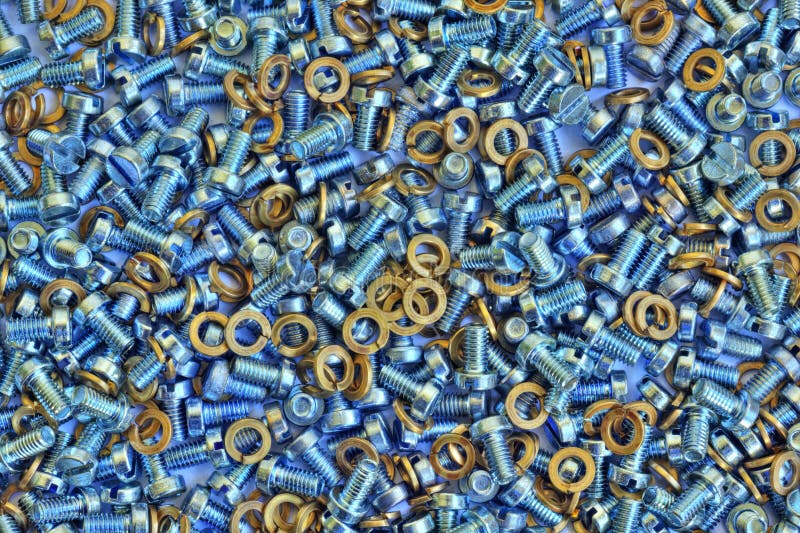Metal background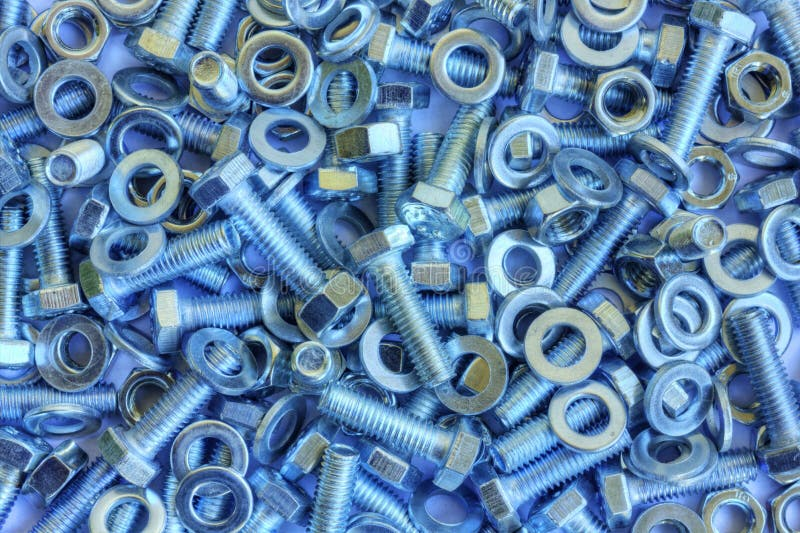Metal background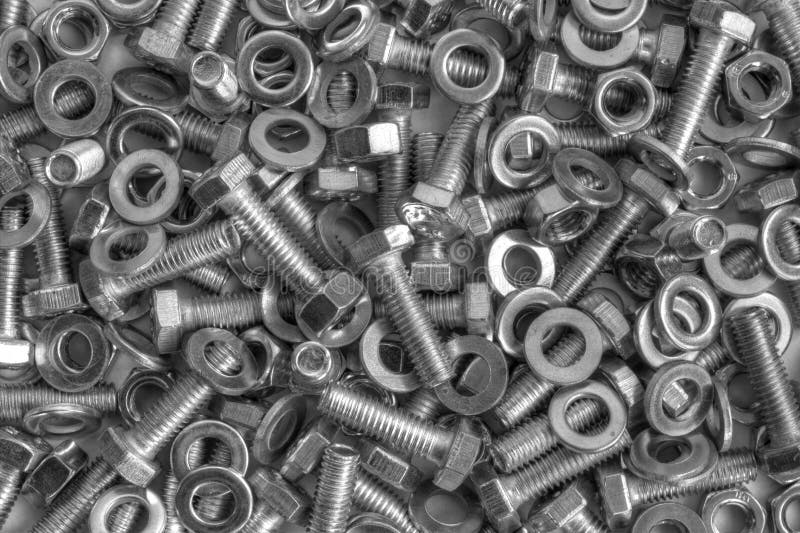Metal background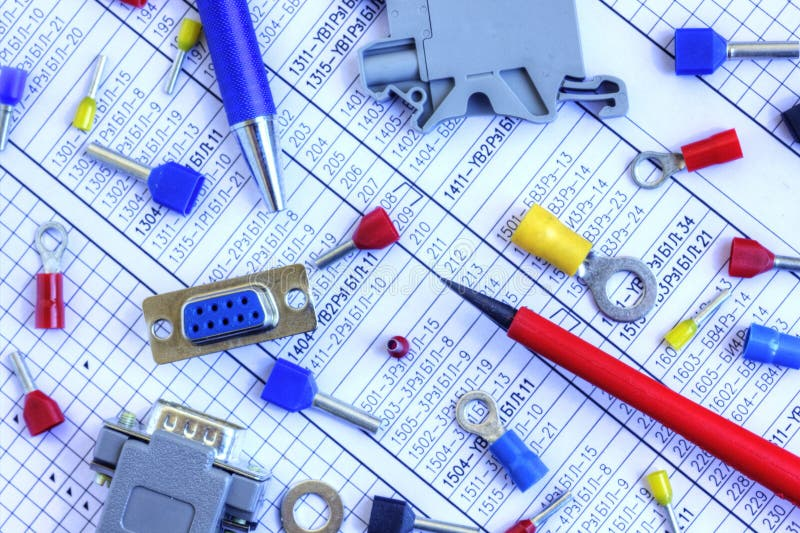Electrical components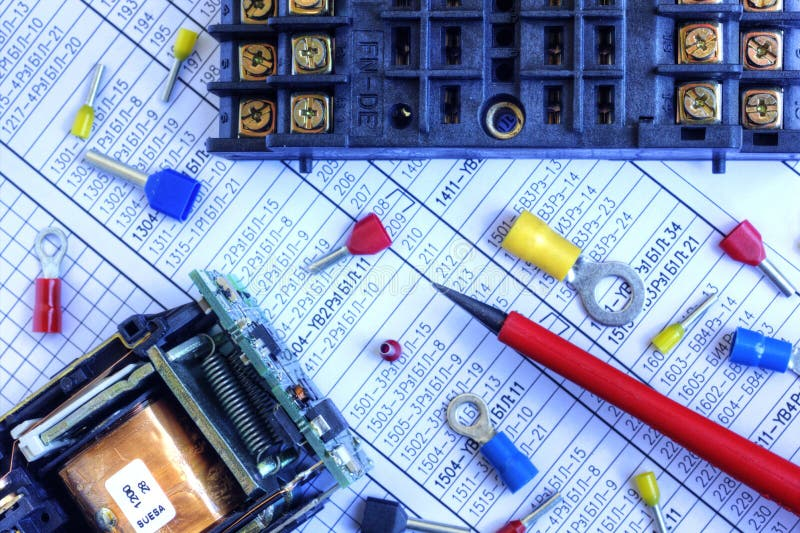Electrical components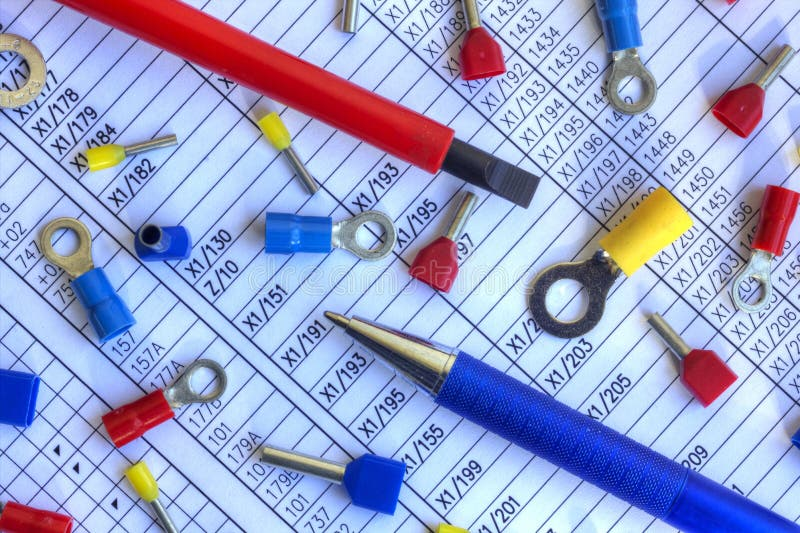Electrical parts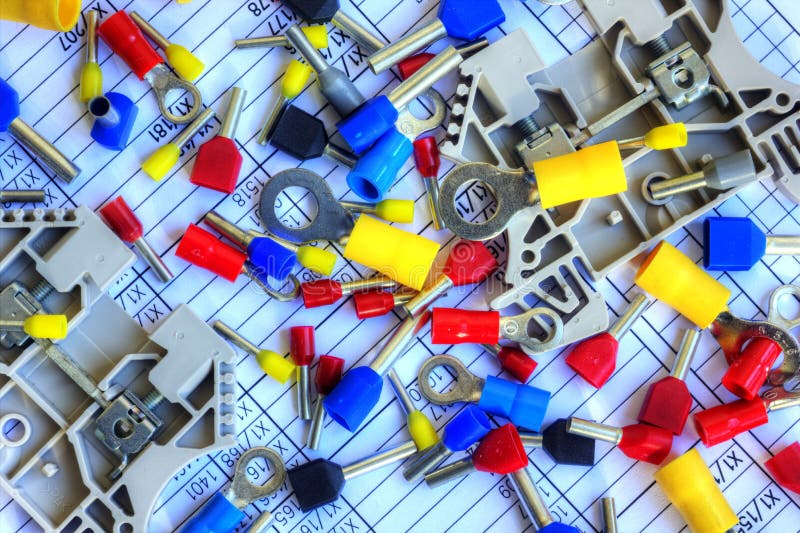Electrical components